Next: Solution of electron equations Up: Particle-in-cell codes Previous: Introduction

## Normalization scheme

It is convenient to normalize time with respect to, where(294)

is the so-called plasma frequency: i.e., the typical frequency of electrostatic electron oscillations. Likewise it is convenient to normalize length with respect to the so-called Debye length: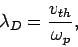which is the length-scale above which the electrons exhibit collective (i.e., plasma-like) effects, instead of acting like individual particles.

Our normalized equations take the form: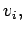(295)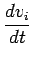(296)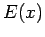(297)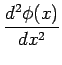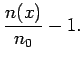(298)

whereas our initial distribution function becomes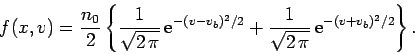(299)

Note that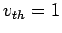in normalized units.

Let us solve the above system of equations in the domain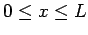. Furthermore, for the sake of simplicity, let us adopt periodic boundary conditions: i.e., let us identify the left and right boundaries of our solution domain. It follows that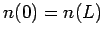,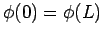, and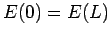. Moreover, any electron which crosses the right boundary of the solution domain must reappear at the left boundary with the same velocity, and vice versa.Next: Solution of electron equations Up: Particle-in-cell codes Previous: Introduction
Richard Fitzpatrick 2006-03-29